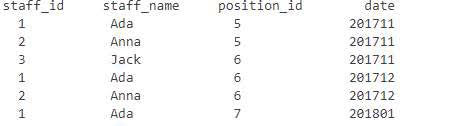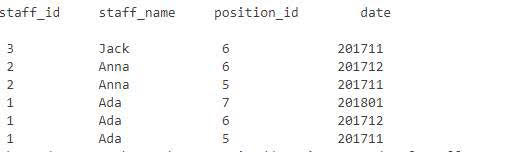1 ada 7 201801和 2 anna 6 201712

3 jack 6 201711
2 anna 6 201712
2 anna 5 201711

List resultListOrder=...

for(Object[] rowOrder:resultListOrder)
{
for(Object[] row:resultListOrder)
{
//staff_id相同的且date较大
if((int)rowOrder ==(int) row&&(int)rowOrder>(int)row)
{
Object[] tempRow = row;
//remove date较小记录
resultListOrder.remove(resultListOrder.indexOf(row));
//将date较小记录插入较大记录下一行

``````        }
}
``````

}

Iterator，但是不知道怎么把刚刚那个算法改成Iterator，请问该怎么修改能实现正确的排序

9个回答lvgo 用手机回复的。卡了。。。不好意思回复了那么多条
2 年多之前 回复

List list = new ArrayList();
public void sort(){
Iterator itr = list.iterator();
while(itr.hasNext()){
itr.remove();
}
}RunWithSmile 回复wuzangji0642: 你用if条件包裹itr.remove();当满足条件才删除。实在不行就换成最原始的for（；；）就不会产生这样的错误了
2 年多之前 回复wuzangji0642 Iterator不能在指定位置remove,我要通过if条件判断才能找到需要改变位置的那条记录，hasnext这个没法用吧
2 年多之前 回复
`````` public class TestListSort {
public static void main(String[] args) throws ParseException {
SimpleDateFormat sdf = new SimpleDateFormat("yyyyMM");
List<Student> list = new ArrayList<Student>();
Student s1 = new Student();
s1.setId(1);
s1.setPostionId(5);
s1.setEntryDate(sdf.parse("201711"));

Student s2 = new Student();
s2.setId(2);
s2.setName("Anna");
s2.setPostionId(5);
s2.setEntryDate(sdf.parse("201711"));

Student s3 = new Student();
s3.setId(3);
s3.setName("Jack");
s3.setPostionId(6);
s3.setEntryDate(sdf.parse("201711"));

Student s6 = new Student();
s6.setId(1);
s6.setPostionId(6);
s6.setEntryDate(sdf.parse("201712"));

Student s4 = new Student();
s4.setId(2);
s4.setName("Anna");
s4.setPostionId(6);
s4.setEntryDate(sdf.parse("201712"));

Student s5 = new Student();
s5.setId(1);
s5.setPostionId(7);
s5.setEntryDate(sdf.parse("201801"));
System.out.println("before sort..........................");
list.forEach(item ->{
System.out.println(item);
});

list.sort(new Comparator<Student>() {
@Override
public int compare(Student o1, Student o2) {
if(o1.getId()==o2.getId()) {
Long time1 = o1.getEntryDate().getTime();
Long time2 = o2.getEntryDate().getTime();
return time2.compareTo(time1);
}
return o2.getId().compareTo(o1.getId());
}

});
System.out.println("after sort..........................");
list.forEach(item ->{
System.out.println(item);
});
}
}
``````

Iterator iterator = resultListOrder.iterator() ;
while(iterator.hasNext()){
Object[] b = (Object[]) iterator.next();

``````}
``````

ListIterator it = arrayList.listIterator();

// while(it.hasNext())
// {

// String item = it.next();

// if(item.toLowerCase().indexOf(s.toLowerCase()) == 0)
// {

// //it.remove();
// arrayList.remove(item); //这里删除后，在下一次迭代的时候就会出BUG，用for循环
// }

// }

//

for (int i = 0 ; i < arrayList.size() ; i ++) {
if(arrayList.get(i).toLowerCase().indexOf(s.toLowerCase()) == 0)
{
String item = arrayList.get(i);
arrayList.remove(i);